Technical Article

# Simple Priority Arbiters: Allocating Resources in Embedded Systems with VHDL and Logisim

February 11, 2019 by Stephen St. Michael

## This article looks at the use cases and benefits of the arbiter with an implementation of a simple priority arbiter in VHDL.

This article looks at the use cases and benefits of the arbiter with an implementation of a simple priority arbiter in VHDL.

Arbitration is an important part of any modern computer system. From bus arbitration in communication protocols like I2C and CAN, to memory arbitration in multi-processor systems, arbiters can be found anywhere a resource needs to be shared.

Arbiters can be synchronous (i.e., clocked) or asynchronous, and they work by inputting requests and granting access to resources based on those requests.

In the embedded world, resources are always limited. Using an arbiter can simplify resource control and add priority to competing subsystems, all while increasing both system performance and robustness.

### The Basics

#### What Is an Arbiter?

At its most basic, an arbiter is a device that takes as input N requests, and outputs a single grant, in the form of a one-hot. A one-hot is a group of bits of arbitrary size consisting of all zeros except for one; i.e., one bit is logic high, or “hot.” In this way, the arbiter looks at its set of inputs and allows a single device access to the resource.

#### Example of an Arbiter in Action

Let's say we have three devices each with a request signal tied to an arbiter. “Device” here is used as a general term for any requester. The requester could be a FIFO (first-in, first-out) queue, a CPU, a state machine, etc. When the device needs access to a resource, it simply sets its request signal high. The arbiter checks its inputs and grants access.

Figure 1 shows a block diagram with a truth table below it. In this example, the grant signal is not used in any specific way. How the grant signal gives access to the resource depends on the application and implementation.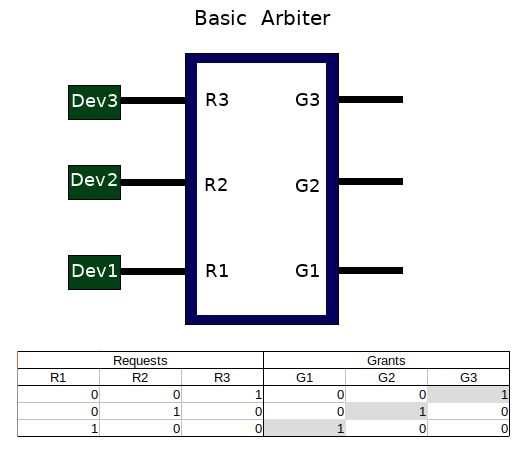##### Figure 1. Arbiter with request and grant signals

Figure 1 depicts the case when each device independently makes a request.

But what about when two or more devices make a request simultaneously? To solve this problem, we can build priority into our arbiter.

#### How to Build Priority into an Arbiter

Figure 2 shows a modified truth table that gives priority based on request. Dev3, with request R3, has priority over Dev2 (with R2) and Dev1 (with R1). Dev2, with request R2, has priority over Dev1 (with R1). Notice again that the grant output is always a one-hot. This is essential for keeping multiple devices from gaining access to the same resource at the same time.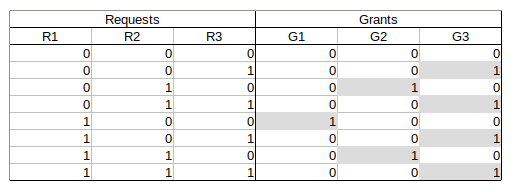### Implementing Arbiters in Design

Now we know the basic idea behind the arbiter and what the logical behavior is. From here, we can begin implementing our design.

There are different ways to implement the same functionality. You could simplify the truth table above using a Karnaugh map or begin your implementation in a hardware description language.

I personally like to draw the logic diagram if it is not overly complicated. The ability to visualize the logic gates along with the truth table only makes it easier when it comes time to build.

#### Arbiter in Logisim

Logisim is an open-source logic simulator for digital designers, hobbyists, and students. It is completely graphical and enables the user to build and test simple designs using all of the everyday logic blocks including AND gates, multiplexers, adders, flip-flops, registers, and even random access memory (RAM).

Figure 3 and Figure 4, below, show a three-bit priority arbiter. The tags with letters 'a' and 'b' are called tunnels and signify that points with the same letter are connected even though a physical wire is not drawn between them. The bright green paths represent logic high or TRUE signals.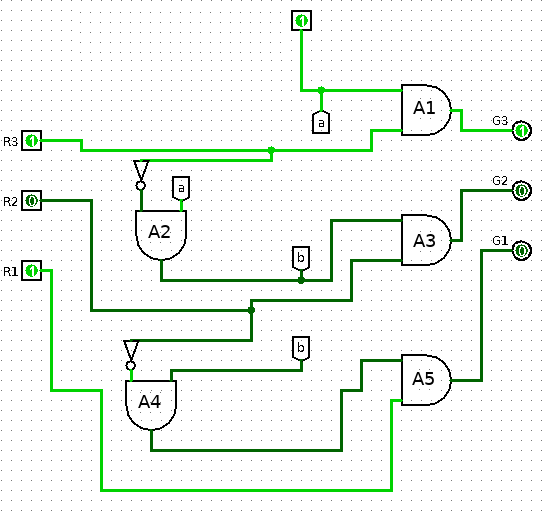##### Figure 3. A three-input arbiter simulated in Logisim

For starters, notice that the first input of the uppermost AND gate (A1) is held high. This logic high gets ANDed at A2, with the complement of request R3. The output of A2 gets ANDed at A4, with the complement of request R2. The output of A4 then determines if request R1 gets granted.

In this way, you can see that this initial logic high is rippled through the arbiter. If any request goes high, its complement is passed down the arbiter, ensuring any requests below it are not granted. This is how we create an arbiter's priority. Figure 3 shows that even though the arbiter is receiving two requests, R1 and R3, only a single grant is output (G3).

Figure 4 shows a similar situation, except now it's request R1 and request R2 competing for access to a resource.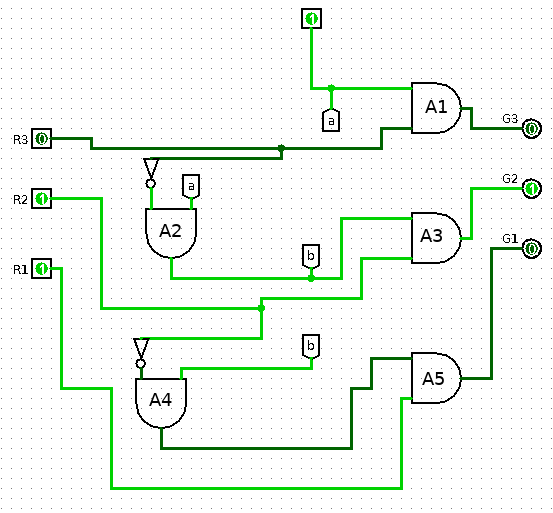##### Figure 4

Request R2 has a higher priority than R1, therefore the grant signal that goes high is G2. We can see that this high priority comes from the fact that request R2 is complemented at gate A4, keeping the output of A5 (i.e., grant G1) from going high.

Simulating small logic diagrams, like those above, are great for a quick proof of concept. When something large needs to be built a more sophisticated tool ought to be used.

#### Arbiter in VHDL

To implement our arbiter in VHDL, I used Xilinx ISE. Other tools like Quartus II or Active-HDL would work, as well.

Listing 1, below, shows an implementation of a three-input priority arbiter.

-- Listing 1: Arbiter

library IEEE;
use IEEE.STD_LOGIC_1164.ALL;

entity arbiter is
port(
R: in STD_LOGIC_VECTOR(3 downto 1);
G: out STD_LOGIC_VECTOR(3 downto 1));

end arbiter;

architecture grant of arbiter is
begin

G <= "100" when R(3) = '1' else
"010" when R(3) = '0' and R(2) = '1' else
"001" when R(3) = '0' and R(2) = '0' and R(1) = '1' 	else "000";

end grant;
##### Listing 1. Arbiter implemented in VHDL

Notice the use of std_logic_vector in the entity block to build the request and grant ports.

The R vector represents the three input requests and G is used for the output grants. As far as the architecture goes, we're only using combinational logic, which is why you don't see any processes.

The first line, once the architecture begins, sets G to '100' when R(3) is high, ignoring the rest of the inputs. Next, if R(3) is low then we check R(2) and set G equal to '010' if R(2) is high. Notice we still ignore R(1). If both R(3) and R(2) are low, we do check R(1). If R(1) is high, G gets '001'. Lastly, we use the else statement to ensure that G is set to '000' if all inputs are low. This is a simple way to implement our priority scheme in VHDL.

#### Testing a VHDL Arbiter in Testbench

Now to test our code. Listing 2 shows a simple testbench that we can use to instantiate and test our new design.

-- Listing 2: Arbiter TestBench

library ieee;
use ieee.std_logic_1164.all;

entity arbiterTest is
end arbiterTest;

architecture behavior of arbiterTest is

-- Component Declaration for the Unit Under Test (UUT)
component arbiter
port(
R : in  std_logic_vector(3 downto 1);
G : out  std_logic_vector(3 downto 1)
);
end component;

--Inputs
signal r : std_logic_vector(3 downto 1) := (others => '0');

--Outputs
signal g : std_logic_vector(3 downto 1);

begin

-- Instantiate the Unit Under Test (UUT)
uut: arbiter port map (
r => R,
g => G
);

-- Stimulus process
stim_proc: process
begin

r <= "000";

wait for 1 ns;
r <= "001";

wait for 1 ns;
r <= "010";

wait for 1 ns;
r <= "011";

wait for 1 ns;
r <= "100";

wait for 1 ns;
r <= "101";

wait for 1 ns;
r <= "110";

wait for 1 ns;
r <= "111";

wait for 1 ns;
r <= "000";
wait;

end process;

end;
##### Listing 2. Arbiter testbench implemented in VHDL

While testbench design is outside the scope of this article, a brief explanation follows.

Essentially, I declare the arbiter component along with test signals, r and g. Then I instantiate the arbiter component as my unit under test and map the test signals to its ports. I then run through all eight input combinations, waiting a nanosecond between each one. I've ordered these to match the truth table from Figure 2. The last part of the testbench sets the inputs equal to '000' and then waits forever. While there are more elegant ways to test all input combinations, this appeared to be the simplest and most straightforward implementation.

To see how the arbiter behaves within this testbench, I simulated the testbench in ISE and produced a timing diagram, shown in Figure 5.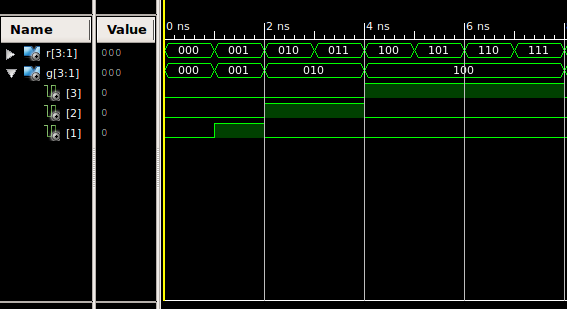##### Figure 5. Arbiter testbench timing diagram

The top bar is our request vector while the second bar is the grant vector.

This grant vector has been expanded so that we can see G3, G2, and G1. Notice that when request R3 (the most significant bit of the request vector) is high, grant G3 is also high, regardless of the state of the other inputs. When request R2 is high and R3 is low, G2 is high. G1 is high only when all requests except for R1 are low. Through this timing diagram, we can see that our arbiter is behaving as expected.

The simple priority arbiter is, as its name suggests, simple. There are better, more efficient ways to implement an arbiter. One problem with the priority arbiter is that low-priority requests may never be granted. One way this can be solved is with a round-robin arbiter, which gives each requester access to the resource for a short time. A round-robin and priority arbiter can be combined to get the best of both implementations. These designs may be explored in a future article.

### Conclusion

This article introduced the arbiter, with an implementation of a simple priority arbiter in VHDL. The arbiter is a mediator between different system components and system resources. This could be two CPU cores that need to access shared memory, or two microcontrollers trying to take control of a communication bus. Whatever the application, the arbiter is a low-cost and relatively simple solution to an often complex problem.